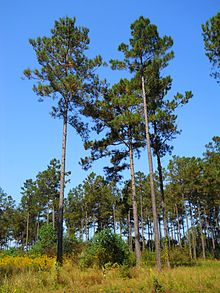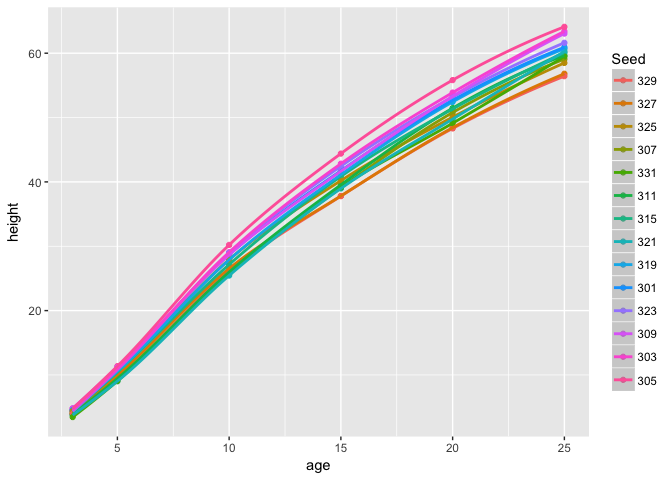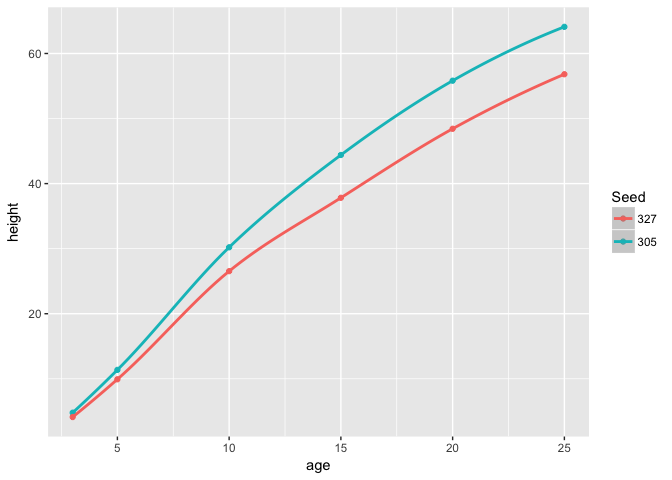## Introduction to R markdown - 1

Last update on April 27, 2017.

Tree growth analysis

This is an R Markdown Notebook. When you execute code within the notebook, the results appear beneath the code.Photo of a stand of Loblolly pine

## Introduction

In this exercise we will be working with data that is already part of your R installation. The Loblolly pine data can be loaded using data(“Loblolly”).

library(ggplot2)
library(dplyr)

data("Loblolly")
head(Loblolly)
##    height age Seed
## 1    4.51   3  301
## 15  10.89   5  301
## 29  28.72  10  301
## 43  41.74  15  301
## 57  52.70  20  301
## 71  60.92  25  301
unique(levels(Loblolly\$Seed))
##   "329" "327" "325" "307" "331" "311" "315" "321" "319" "301" "323"
##  "309" "303" "305"

Let’s visualize the data using ggplot2

ggplot(Loblolly) + geom_point(aes(x=age, y=height, colour=Seed)) + stat_smooth(aes(x=age, y=height, colour=Seed))ggplot(Loblolly %>% filter(Seed == 305 | Seed == 327)) + geom_point(aes(x=age, y=height, colour=Seed)) + stat_smooth(aes(x=age, y=height, colour=Seed))# Filtering by Seed source/type

### Pingbacks

Pingbacks are closed.